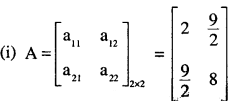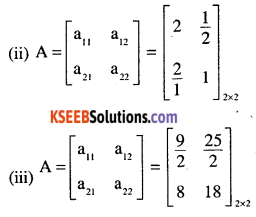# 2nd PUC Maths Question Bank Chapter 3 Matrices Ex 3.1

Students can Download Maths Chapter 3 Matrices Ex 3.1 Questions and Answers, Notes Pdf, 2nd PUC Maths Question Bank with Answers helps you to revise the complete Karnataka State Board Syllabus and score more marks in your examinations.

## Karnataka 2nd PUC Maths Question Bank Chapter 3 Matrices Ex 3.1

### 2nd PUC Maths Matrices NCERT Text Book Questions and Answers Ex 3.1

Question 1.
In the matrix
$$\mathbf{A}=\left[\begin{array}{rrrr}{2} & {5} & {19} & {-7} \\{35} & {-2} & {\frac{5}{2}} & {12} \\{\sqrt{3}} & {1} & {-5} & {17}\end{array}\right]$$
(i) The order of the matrix,
(ii) The number of elements,
(iii) Write the elements a13, a21, a33, a24 ,a23,
(i) The order of the matrix is 3 x 4
(ii) Number of elements = 12
(iii) a13= 19
a21 = 35
a33 = -5
a24 = 12
a23 = $$\frac{5}{2}$$

Question 2.
If a matrix has 24 elements, what are the possible orders it can have? What, if it has 13 elements?
1 x 24; 24 x 1; 2 x 12;  12 x 2;
3 x 8; 8 x 3; 4 x 6; 6 x 4
13 elements
1 x 13;   13 x 1Question 3.
If a matrix has 18 elements, what are the possible orders it can have? What, if it has 5 elements?
18 elements
1 x 18;      18 x 1;      2 x 9;      9 x 2;       3 x 6;  6 x 3
5 elements
1 x 5;    5 x 1;

Question 4.
Constract a 2 x 2 matrix A =[aij] ,whose element are given by:
$$a_{u}=\frac{(i+j)^{2}}{2}$$
$$a_{i j}=\frac{i}{j}$$
$$a_{i j}=\frac{(i+2 j)^{2}}{2}$$Question 5.
Construct a 3 x 4 matrix, whose elements are given by:
(i) $$a_{i j}=\frac{1}{2}|-3 i+j|$$
(ii) $$a_{i j}=2 i-j$$Question 6.
Find the values of x, y and z from the following equations:
$$\text { (i) }\left[\begin{array}{ll}{4} & {3} \\{x} & {5}\end{array}\right]=\left[\begin{array}{ll}{y} & {z} \\{1} & {5}\end{array}\right]$$
x = 1, y = 4, z = 3

(ii) $$\left[\begin{array}{cc}{x+y} & {2} \\{5+z} & {xy}\end{array}\right]=\left[\begin{array}{cc}{6} & {2} \\{5} & {8}\end{array}\right]$$
5 + z = 5 = $$\succ$$– z = 0
x + y = 6 = $$\succ$$ x = 2
xy = 8 = $$\succ$$ x = 4
y = 4
4 = 2(iii) $$\left[\begin{array}{r}{\mathbf{x}+\mathbf{y}+\mathbf{z}} \\{\mathbf{x}+\mathbf{z}} \\{\mathbf{y}+\mathbf{z}}\end{array}\right]=\left[\begin{array}{l}{\mathbf{9}} \\{\mathbf{5}} \\{\mathbf{7}}\end{array}\right]$$
a – b = -1
2a – b = 0
a = 1
∴ 2a – b = 0
⇒ b = 2
2a + c = 5
c = 5 – 2 = 3 ∴ c = 3
d= 13-9 = 4
a = 1, b = 2 ,c = 3, d = 4

Question 8.
A = [aij]m x n\ is a square matrix, if
(A) m < n
(B) m>n
(C) m = n
(D) None of these
(C) m = n as in a square matrix, the number of rows = number of colums.Question 9.
Which of the given values of x and y make the following pair of matrices equal
$$\left[\begin{array}{cc}{3 x+7} & {5} \\{y+1} & {2-3 x}\end{array}\right],\left[\begin{array}{cc}{0} & {y-2} \\{8} & {4}\end{array}\right]$$
(A) $$x=\frac{-1}{3}, y=7$$
(B) Not possible to find
(C) $$y=7, x=\frac{-2}{3}$$
(D) $$x=\frac{-1}{3}, y=\frac{-2}{3}$$